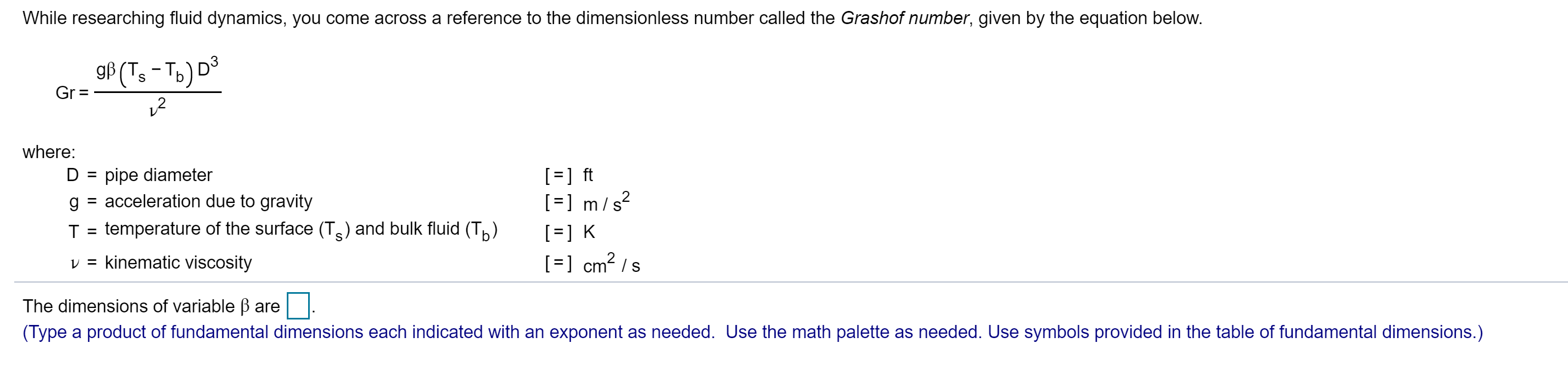# While researching fluid dynamics, you come across a reference to the dimensionless number called the Grashof number, given by the equation below. gf (T,-To) D S Gr = where: ] ft ]m/ s D pipe diameter acceleration due to gravity g temperature of the surface (Ts) and bulk fluid (T) T K cm2 s v = kinematic viscosity The dimensions of variable B are (Type a product of fundamental dimensions each indicated with an exponent as needed. Use the math palette as needed. Use symbols provided in the table of fundamental dimensions.)

Question

What are the dimensions for the variable beta given the following?help_outlineImage TranscriptioncloseWhile researching fluid dynamics, you come across a reference to the dimensionless number called the Grashof number, given by the equation below. gf (T,-To) D S Gr = where: ] ft ]m/ s D pipe diameter acceleration due to gravity g temperature of the surface (Ts) and bulk fluid (T) T K cm2 s v = kinematic viscosity The dimensions of variable B are (Type a product of fundamental dimensions each indicated with an exponent as needed. Use the math palette as needed. Use symbols provided in the table of fundamental dimensions.) fullscreen

1 Rating

### Want to see this answer and more?

Experts are waiting 24/7 to provide step-by-step solutions in as fast as 30 minutes!*

*Response times vary by subject and question complexity. Median response time is 34 minutes and may be longer for new subjects.
Tagged in
SciencePhysics

### Other